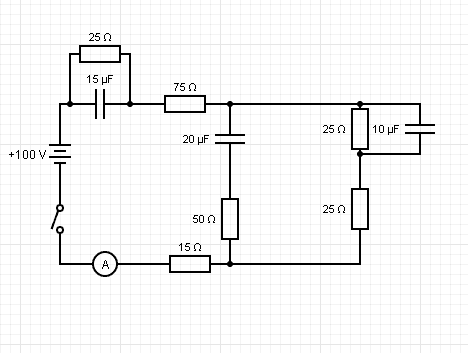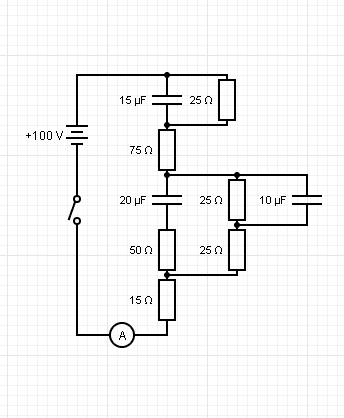# Potential difference in Capacitors

• jisbon
In summary, after a long time the capacitor reaches its P.D. and it's safe to assume the equation looks like: ##2=15.15e^{\frac {-t}{RC}}##

#### jisbon

Homework Statement
Consider the circuit below. The switch is closed for a long time, then is opened. Calculate the time taken for ##15\mu F## capacitor to reach P.D of 2V.
Relevant Equations
##Q(t) =Q_{0}e^{\frac {-t}{RC}}##
Firstly, I'm given this complicated circuit as shown below.What I have to do first, is to simplify it, which I will need help in checking.One question here: It's not possible to simplify this by adding resistors in series and capacitors in series am I, right? Or is it possible in this case?

So if I'm right, the next step to do find the charge in the ##15\mu## F capacitor after a long time, which is capacitance * voltage, which is presumably be 15V in this case? Hence ##Q_{0}## = ##15\mu## F * 100V ?

So for it to reach P.D of 2V, I will have to calculate the charge at that point. Since charge = capacitance * voltage, my charge at that point will be ##15\mu## * 2V = ##30*10^-6##
I will then use this formula where: ##Q(t) =Q_{0}e^{\frac {-t}{RC}}##
## 30*10^-6=(15*10^-6)(100)e^{\frac {-t}{RC}}##
Will R in this case be 25?

Thanks.

jisbon said:
Homework Statement: Consider the circuit below. The switch is closed for a long time, then is opened. Calculate the time taken for ##15\mu F## capacitor to reach P.D of 2V.
Homework Equations: ##Q(t) =Q_{0}e^{\frac {-t}{RC}}##

Firstly, I'm given this complicated circuit as shown below.
View attachment 250207
What I have to do first, is to simplify it, which I will need help in checking.

View attachment 250208
One question here: It's not possible to simplify this by adding resistors in series and capacitors in series am I, right? Or is it possible in this case?
No. What does it mean that two elements of circuit are connected in series? Can a third element be connected to the terminals in common?
jisbon said:
So if I'm right, the next step to do find the charge in the ##15\mu## F capacitor after a long time, which is capacitance * voltage, which is presumably be 15V in this case? Hence ##Q_{0}## = ##15\mu## F * 100V ?
Why should be the voltage 15 V or100 V across the ##15\mu## F capacitor?
Think: does any current flow through the capacitors when the switch is closed for very long time ? And what is the voltage across the resistor connected in parallel with the capacitor?
jisbon said:
So for it to reach P.D of 2V, I will have to calculate the charge at that point. Since charge = capacitance * voltage, my charge at that point will be ##15\mu## * 2V = ##30*10^-6##
I will then use this formula where: ##Q(t) =Q_{0}e^{\frac {-t}{RC}}##
## 30*10^-6=(15*10^-6)(100)e^{\frac {-t}{RC}}##
Will R in this case be 25?

Thanks.

••jisbon and Delta2
In the so called "steady state" of a DC-circuit ( and we are in the steady state when the statements says things like "the circuit is closed for a very long time" which means time big enough to reach the steady state) the capacitors are like they don't exist.
So first step is to remove all capacitors and replace them with open switches. Then calculate all the voltages and currents of interest. In this steady state the voltage of a capacitor would be the voltage of the opened switch with which we replaced the capacitor.

Then when the switch is opened , we replace back the capacitors and check which are the only closed loops now.
Then calculate voltages and currents of interest on these closed loops.

Last edited:
•jisbon
Nope after a very long time, it's basically a open circuit
Delta2 said:
In the so called "steady state" of a DC-circuit ( and we are in the steady state when the statements says things like "the circuit is closed for a very long time" which means time big enough to reach the steady state) the capacitors are like they don't exist.
So first step is to remove all capacitors and replace them with open switches. Then calculate all the voltages and currents of interest. In this steady state the voltage of a capacitor would be the voltage of the opened switch with which we replaced the capacitor.

Then when the switch is opened , we replace back the capacitors and check which are the only closed loops now.
Then calculate voltages and currents of interest on these closed loops.
Following step by step with regards on what you have stated:

I have calculated the current to be 0.606A after a long time it was charged.
Hence voltage in 25-ohm resistor (since it is parallel to the 15F capacitor) = 0.606 *25 = 15.15V
So is it safe to assume the equation now looks like:
##2=15.15e^{\frac {-t}{RC}}##

PS: I got the answer I was looking for. Thanks!

•Delta2